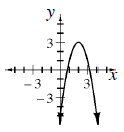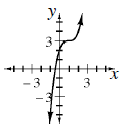### Home > A2C > Chapter 8 > Lesson 8.1.4 > Problem8-59

8-59.

Sketch a graph of each equation below.

1. $y = −2\left(x − 2\right)^{2} + 3$1. $y = \left(x − 1\right)^{3} + 3$